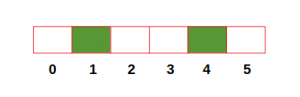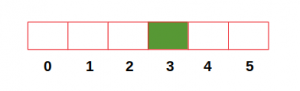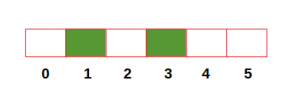# Find maximum distance between any city and station

Given the number of cities n numbered from 0 to n-1 and the cities in which stations are located, the task is to find the maximum distance between any city and its nearest station. Note that the cities with stations can be given in any order.

Examples:

```Input: numOfCities = 6, stations = [1, 4]
Output: 1

Input: numOfCities = 6, stations = 
Output: 3

Input: numOfCities = 6, stations = [3, 1]
Output: 2
```

## Recommended: Please try your approach on {IDE} first, before moving on to the solution.

1. The below figure represents the first example containing 6 cities and the cities with stations highlighted with green color. In this case, the farthest cities from their nearest stations are 0, 2, 3 and 5 each at a distance of 1. Hence maximum distance is 1.2. In the second example, the farthest city from its nearest station is 0 which is at a distance of 3. Hence maximum distance is 3.3. In the third example, the farthest city from its nearest station is 5 which is at a distance of 2. Hence maximum distance is 2.Approach: There are three possible cases in this problem:

1. When the farthest city is between two stations.
2. When the farthest city is on the left side of the first station.
3. When the farthest city is on the right side of the last station.

Below is the algorithm to solve the above problem:

• Initialize a boolean array of size n (number of cities) with False. Then mark the values of cities with stations as True
• Initialize a variable dist with 0. Initialize another variable maxDist with value which is equal to the first city with station (used for case 2).
• Start looping through all the cities ony by one.
• If the current city has a station, then assign the maximum of (dist+1)//2 and maxDist to maxDist (used for case 1). Also, assign 0 to dist.
• Else, increment dist.
• At the end, return the maximum of dist and maxDist (used for case 3).

Below is the implementation of the above approach:

## C++

 `// C++ program to calculate the maximum  ` `// distance between any city ` `// and its nearest station ` `#include ` ` `  `using` `namespace` `std; ` ` `  `// Function to calculate the maximum  ` `// distance between any city and its nearest station ` `int` `findMaxDistance(``int` `numOfCities,``int` `station[],``int` `n) ` `{  ` `    ``// Initialize boolean list ` `    ``bool` `hasStation[numOfCities + 1] = {``false``}; ` `     `  `    ``// Assign True to cities containing station ` `    ``for` `(``int` `city = 0; city < n; city++) ` `    ``{ ` `        ``hasStation[station[city]] = ``true``; ` `    ``} ` `         `  `    ``int` `dist = 0; ` `    ``int` `maxDist = INT_MAX; ` ` `  `    ``for``(``int` `i = 0; i < n; i++) ` `    ``{ ` `        ``maxDist = min(station[i],maxDist); ` `    ``} ` ` `  `    ``for` `(``int` `city = 0; city < numOfCities; city++) ` `    ``{ ` `        ``if` `(hasStation[city] == ``true``) ` `        ``{ ` `            ``maxDist = max((dist + 1) / 2, maxDist); ` `            ``dist = 0; ` `        ``} ` `        ``else` `            ``dist += 1; ` `    ``} ` `    ``return` `max(maxDist, dist); ` `}  ` ` `  `//Driver code ` `int` `main() ` `{  ` `    ``int` `numOfCities = 6; ` `    ``int` `station[] = {3, 1}; ` `    ``int` `n = ``sizeof``(station)/``sizeof``(station); ` ` `  `    ``cout << ``"Max Distance:"` `<< findMaxDistance(numOfCities, ` `                                                ``station, n); ` `} ` ` `  `//This code is contributed by Mohit Kumar 29 `

## Java

 `// Java program to calculate the maximum  ` `// distance between any city ` `// and its nearest station ` `import` `java.util.*; ` ` `  `class` `GFG ` `{ ` ` `  `// Function to calculate the maximum  ` `// distance between any city and its nearest station ` `static` `int` `findMaxDistance(``int` `numOfCities, ` `                            ``int` `station[],``int` `n) ` `{  ` `    ``// Initialize boolean list ` `    ``boolean` `hasStation[] = ``new` `boolean``[numOfCities + ``1``]; ` `     `  `    ``// Assign True to cities containing station ` `    ``for` `(``int` `city = ``0``; city < n; city++) ` `    ``{ ` `    ``hasStation[station[city]] = ``true``; ` `    ``} ` `         `  `    ``int` `dist = ``0``; ` `    ``int` `maxDist = Integer.MAX_VALUE; ` ` `  `    ``for``(``int` `i = ``0``; i < n; i++) ` `    ``{ ` `        ``maxDist = Math.min(station[i],maxDist); ` `    ``} ` ` `  `    ``for` `(``int` `city = ``0``; city < numOfCities; city++) ` `    ``{ ` `        ``if` `(hasStation[city] == ``true``) ` `        ``{ ` `            ``maxDist = Math.max((dist + ``1``) / ``2``, maxDist); ` `            ``dist = ``0``; ` `        ``} ` `        ``else` `            ``dist += ``1``; ` `    ``} ` `    ``return` `Math.max(maxDist, dist); ` `}  ` ` `  `//Driver code ` `public` `static` `void` `main(String args[]) ` `{  ` `    ``int` `numOfCities = ``6``; ` `    ``int` `station[] = {``3``, ``1``}; ` `    ``int` `n = station.length; ` ` `  `    ``System.out.println(``"Max Distance:"``+ ` `        ``findMaxDistance(numOfCities,station, n)); ` `} ` `} ` ` `  `// This code is contributed by ` `// Surendra_Gnagwar `

## Python

 `# Python3 code to calculate the maximum  ` `# distance between any city and its nearest station ` ` `  `# Function to calculate the maximum  ` `# distance between any city and its nearest station ` `def` `findMaxDistance(numOfCities, station): ` `     `  `    ``# Initialize boolean list ` `    ``hasStation ``=` `[``False``] ``*` `numOfCities ` `    ``# Assign True to cities containing station ` `    ``for` `city ``in` `station: ` `        ``hasStation[city] ``=` `True` `         `  `    ``dist, maxDist ``=` `0``, ``min``(station) ` ` `  `    ``for` `city ``in` `range``(numOfCities): ` `        ``if` `hasStation[city] ``=``=` `True``: ` `            ``maxDist ``=` `max``((dist ``+` `1``) ``/``/` `2``, maxDist) ` `            ``dist ``=` `0` `             `  `        ``else``: ` `            ``dist ``+``=` `1` `             `  `    ``return` `max``(maxDist, dist) ` `     `  `numOfCities ``=` `6` `station ``=` `[``3``, ``1``] ` `print``(``"Max Distance:"``, findMaxDistance(numOfCities, station)) `

## C#

 `// C# program to calculate the maximum  ` `// distance between any city  ` `// and its nearest station  ` `using` `System; ` ` `  `class` `GFG  ` `{  ` ` `  `// Function to calculate the maximum  ` `// distance between any city and its nearest station  ` `static` `int` `findMaxDistance(``int` `numOfCities,  ` `                            ``int` `[]station,``int` `n)  ` `{  ` `    ``// Initialize boolean list  ` `    ``bool` `[]hasStation = ``new` `bool``[numOfCities + 1];  ` `     `  `    ``// Assign True to cities containing station  ` `    ``for` `(``int` `city = 0; city < n; city++)  ` `    ``{  ` `        ``hasStation[station[city]] = ``true``;  ` `    ``}  ` `         `  `    ``int` `dist = 0;  ` `    ``int` `maxDist = ``int``.MaxValue;  ` ` `  `    ``for``(``int` `i = 0; i < n; i++)  ` `    ``{  ` `        ``maxDist = Math.Min(station[i],maxDist);  ` `    ``}  ` ` `  `    ``for` `(``int` `city = 0; city < numOfCities; city++)  ` `    ``{  ` `        ``if` `(hasStation[city] == ``true``)  ` `        ``{  ` `            ``maxDist = Math.Max((dist + 1) / 2, maxDist);  ` `            ``dist = 0;  ` `        ``}  ` `        ``else` `            ``dist += 1;  ` `    ``}  ` `    ``return` `Math.Max(maxDist, dist);  ` `}  ` ` `  `// Driver code  ` `public` `static` `void` `Main(String []args)  ` `{  ` `    ``int` `numOfCities = 6;  ` `    ``int` `[]station = {3, 1};  ` `    ``int` `n = station.Length;  ` ` `  `    ``Console.WriteLine(``"Max Distance:"``+  ` `        ``findMaxDistance(numOfCities,station, n));  ` `}  ` `}  ` ` `  `// This code has been contributed by 29AjayKumar `

## PHP

 ` `

Output:

```Max Distance: 2
```

Time Complexity: O(n)
Space Complexity: O(n)My Personal Notes arrow_drop_upCheck out this Author's contributed articles.

If you like GeeksforGeeks and would like to contribute, you can also write an article using contribute.geeksforgeeks.org or mail your article to contribute@geeksforgeeks.org. See your article appearing on the GeeksforGeeks main page and help other Geeks.

Please Improve this article if you find anything incorrect by clicking on the "Improve Article" button below.